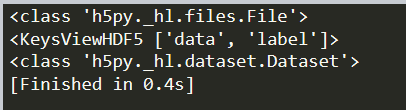# TypeError: 'int' object is not callable

When I use the H5py library to read the .mat dataset, I get the following error. What is wrong? I have not found duplicate function and variable names. Thank you!

``````    tgt_dataset_root = h5py.File('E:\\ADDA\\pytorch-adda-master-lab\\datasets\\lab\\sandy\\mat\\test_target_domain_sandy.mat','r')
data = tgt_dataset_root['data'] # use the key for data here
label = tgt_dataset_root['label'] # use the key for target here

data = numpy.array(data)
label = numpy.array(label)

Could you try to pass the `data` and `label` as tensors using `data = torch.from_numpy(data)`?

Is that so? But the following error also occurs.How do I modify it?

``````    tgt_dataset_root = h5py.File('E:\\ADDA\\pytorch-adda-master-lab\\datasets\\lab\\sandy\\mat\\test_target_domain_sandy.mat','r')
data = tgt_dataset_root['data'] # use the key for data here
label = tgt_dataset_root['label'] # use the key for target here

data = torch.from_numpy(data)
label = torch.from_numpy(label)

tgt_dataset = TensorDataset(data, label)``````

I’m not sure how your data is stored, as the first error message points to an integer scalar value, while it now seems to be a `Dataset` class?
Could you `print(type(data))` after indexing it from `tgt_dataset_root`?

Look at it like this, how do I do next?``````

print (type(dict_data))
print(dict_data.keys())

data = dict_data['data']
print(type(data))``````

You could try to call `data = dict_data['data'].value` to get the underlying numpy array, but I’m not too familiar with h5py.

I got this result after trying. If the data is stored in the Dataset class as you said, how can I operate to load it?

``````    tgt_dataset_root = h5py.File('E:\\ADDA\\pytorch-adda-master-lab\\datasets\\lab\\sandy\\mat\\test_target_domain_sandy.mat','r')
data = tgt_dataset_root['data'].value
label = tgt_dataset_root['label'].value

data = torch.from_numpy(data)
label = torch.from_numpy(label)

tgt_dataset = TensorDataset(data, label)``````

Creating the tensors seems to work, while you are now facing an error stating the data and labels have a different length.
I would recommend to check the correspondence between both tensors, as apparently you have either missing labels or missing data.

Can you help me write down the specific code? I’m a bit confused now. Thank you very much!

One approach would be to get both lengths and cut the longer tensor to the size of the shorter one:

``````min_len = min(data.size(0), target.size(0))
data = data[:min_len]
target = target[:min_len]
``````

However, I would not recommend to just apply this approach, as you are apparently missing some data, so the correspondence between your data and target might be broken.

I encountered such a problem, please take a look.

If you are calling `size` on the numpy arrays, use `.shape` instead.
The provided code should work on the created tensors.

Can you provide specific code, I am not very clear about these conversions. Thank you!

``````tgt_dataset_root = h5py.File('E:\\ADDA\\pytorch-adda-master-lab\\datasets\\lab\\sandy\\mat\\test_target_domain_sandy.mat','r')
data = tgt_dataset_root['data'].value
label = tgt_dataset_root['label'].value

min_len = min(data.shape, label.shape)
data = data[:min_len]
label = label[:min_len]

data = torch.from_numpy(data)
label = torch.from_numpy(label)
``````

should work.

Thank you very much for your patience and guidance. Now both data and label can be printed separately. If it is used as a data set, do we still need to integrate it like this?

``tgt_dataset = TensorDataset(data, label)``

Yes, you still should pass it to a `TensorDataset`.